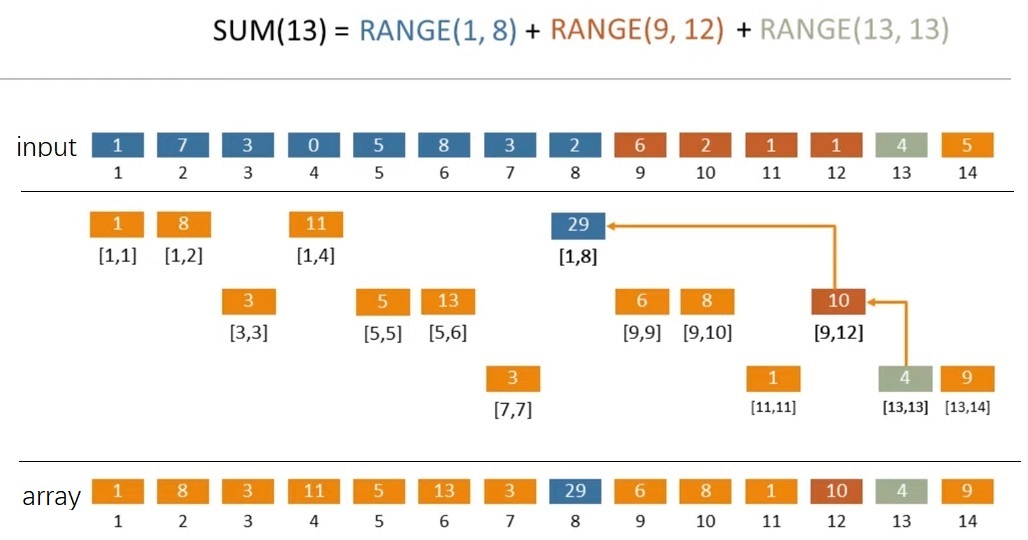# 树状数组（Binary Indexed Tree / Fenwick Tree）学习与实现

1. update(index, delta)：将 delta 加到数组的 index 位置
2. prefix_sum(n)：获取数组的前 n 个元素的和
range_sum(start, end)：获取数组从 [start, end] 的和，相当于 prefix_sum(end) – prefix_sum(start-1)

### 思想

13 = 23 + 22 + 20 = 8 + 4 + 1range(1, 13) = range(1, 8) + range(9, 12) + range(13, 13) = array + array + array

### 前缀和

```range_sum(1, 13) = prefix_sum(13)
= prefix_sum(0b1101)
= array[0b1101] + array[0b1100] + array[0b1000]```

```def _lsb(n: int) -> int:
return n & (-n)

def _prefix_sum(array: list, index: int):
index += 1  # 算法内部，数组从1而不是0开始
result = 0
while index != 0:
result += array[index]
index -= _lsb(index)
return result

def range_sum(array: list, start: int, end: int):
""" 计算数组 [start, end] 闭区间的和 """
return _prefix_sum(array, end) - _prefix_sum(array, start - 1)```

### 更新

```5 = 0b0101
6 = 0b0110
8 = 0b1000```

```def update(array: list, index: int, delta):
index += 1
while index < len(array):
array[index] += delta
index += _lsb(index)```

### Python 实现

```import random

class BinaryIndexedTree:
def __init__(self, init_list: list):
self._array =  * (len(init_list) + 1)
for i, value in enumerate(init_list):
self.update(i, value)

def __len__(self):
""" 内部处理时长度加一，减一后对外部的长度才不变 """
return len(self._array) - 1

@staticmethod
def _lsb(n: int) -> int:
return n & (-n)

def _prefix_sum(self, index: int):
index += 1
result = 0
while index != 0:
result += self._array[index]
index -= self._lsb(index)
return result

def range_sum(self, start: int, end: int):
""" 计算数组 [start, end] 闭区间的和 """
return self._prefix_sum(end) - self._prefix_sum(start - 1)

def update(self, index: int, delta):
index += 1
while index < len(self._array):
self._array[index] += delta
index += self._lsb(index)

if __name__ == "__main__":
MAX = 10000
LENGTH = 1000

test_data = [random.randint(1, MAX) for _ in range(LENGTH)]

binary_indexed_tree = BinaryIndexedTree(test_data)

print(f'the sum of [12, 345] is {sum(test_data[12:346])} (by simple addition)')
print(f'the sum of [12, 345] is {binary_indexed_tree.range_sum(12, 345)} (by binary indexed tree)')

# 随便找10个元素，各加上随机值
for _ in range(10):
random_index = random.randint(0, LENGTH-1)
random_delta = random.randint(1, MAX)
test_data[random_index] += random_delta
binary_indexed_tree.update(random_index, random_delta)

print('\nafter updating some data')
print(f'the sum of [123, 666] is {sum(test_data[123:667])} (by simple addition)')
print(f'the sum of [123, 666] is {binary_indexed_tree.range_sum(123, 666)} (by binary indexed tree)')
```

### Kotlin 实现

```import kotlin.random.Random
import kotlin.random.nextInt

class BinaryIndexedTree(list: List<Int>) {
private val array = MutableList(list.size + 1) { 0 }

init {
for ((i, value) in list.withIndex())
update(i, value)
}

private fun lsb(n: Int) = n and (-n) // bitwise and

private fun prefixSum(index: Int): Int {
var index = index + 1
var result = 0
while (index != 0) {
result += this.array[index]
index -= lsb(index)
}
return result
}

fun rangeSum(start: Int, end: Int) = prefixSum(end) - prefixSum(start - 1)

fun update(index: Int, delta: Int) {
var index = index + 1
while (index < this.array.size) {
this.array[index] += delta
index += lsb(index)
}
}
}

fun main() {
val MAX = 10000
val LENGTH = 1000

val testData = MutableList(LENGTH) { Random.nextInt(1..MAX) }

val binaryIndexedTree = BinaryIndexedTree(testData)

println("the sum of [12, 345] is \${testData.subList(12, 346).reduce { a, b -> a + b }} (by simple addition)")
println("the sum of [12, 345] is \${binaryIndexedTree.rangeSum(12, 345)} (by binary indexed tree)")

// 随便找10个元素，各加上随机值
for (i in 1..10) {
val randomIndex = Random.nextInt(0 until LENGTH)
val randomDelta = Random.nextInt(1..MAX)
testData[randomIndex] += randomDelta
binaryIndexedTree.update(randomIndex, randomDelta)
}

println("\nafter updating some data")
println("the sum of [123, 666] is \${testData.subList(123, 667).reduce { a, b -> a + b }} (by simple addition)")
println("the sum of [123, 666] is \${binaryIndexedTree.rangeSum(123, 666)} (by binary indexed tree)")
}```